1. The composition of every matter comprises of an atom. These atoms have infinitely small structure.
2. In an element, its smallest particle which is an active participator in a chemical reaction is termed as an atom.
3. A substance that cannot be disintegrated into another substance is known as an element.
4. The construction of atom is based on 3 aspects.
• Neutron
• Protons
• Electrons
1. In majority cases related to solid materials,their classification is based on electrical conductivity. Due to this, they may be either,
• Insulators
• Semiconductors
• Conductors
1. For a material to be stated as a conductor, its necessary requirement is to have few mobile electrons which can easily move between atoms. It is only from the valance orbit or outer ring of the atoms that these free electrons can come. Its conductivity depends on electron numbers or quantity that is present in valance

Valence electrons

With the increasing distance between nucleus and electron, its energy level (electrons) also increases. So if you see the last orbit, the energy of the electron is the highest in comparison to electron’s energy in the first orbit. This energy variation follows through each orbit.

These focused electrons having high energy have less bonding with the nucleus resulting in their mobile nature. The mobility regarding last orbit electrons is the reason that they obtain this certain property of merging with other atoms. This combining power in case of last orbit electron related to an atom is stated to be valence electron.

Important points regarding atoms

• Atoms having 4 valence electrons can be stated as semiconductors.
• Atoms having more than 4 valence electrons can be stated as poor conductors.
• Atoms having less than 4 valence electrons can be stated as good conductors

Important data regarding atoms – Electron and Proton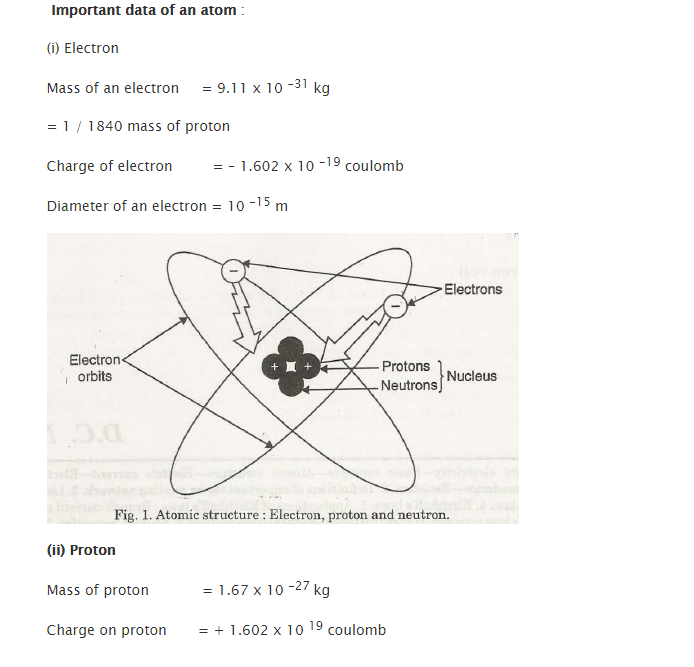Neutron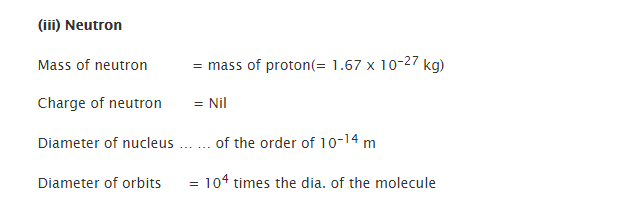In ageneralized sense, the structure of atoms describes to be it electrically neutral. In simplified terms, it can be stated as an atom having a similar number of protons and electrons. Both of these cancel the electrical force of each other.

Contrary to the nature of charges which attract each other, atoms have the tendency to stay together. Proton’s electrical forces do have the hold over electrons in their rings or orbits. Electrical charges which are similar or are alike do repel each other. Due to this, none of the negatively charged electrons collide with each other.

Negative and positive ions

• Loss or gain of an electron from an atom helps it to become an ion.
• Atoms are positively charged on the removal of an electron from a neutral This is known as a positive ion.
• But in case an electron is included in a neutral atom, then that atom is negatively charged. It is known as a negative

1.2. Electric current

A highly controlled movement regarding electron via any substance is known as current.

The movement of electrons at a certain rate is known as current.

Unit of current = 1 ampere

1 ampere = 6.28 x 1018 electrons in a second

This number of electrons passes a certain point in a second.

Coulomb per second = ampere

Charge regarding 6.28 x 1018 electrons is equivalent to 1 coulomb.

1.3. Electromotive force

• A force which is the main reason for the flow of current of electricity is known as electromotive force.
• It is also expressed as e.m.f.
• Potential difference of e.m.f. is denoted by V
• In a circuit between 2 points, V is highlighted as an electrical pressure (r) which is required for driving the current in between them.

Volt

Unit of electromotive force and potential difference is termed as volt. In simplified term it can be expressed as a difference of potential which when across a resistance of one ohm will carry 1 ampere current.

Electron volt

The unit which expresses the energies related to atomic particles is called electron volt. The charge of an electron is depicted via coulomb. When a certain electron whose potential difference is 1 volt is moved through an electric field, the work which is done against a force acts on that charge. The unit of force here is newton.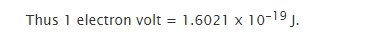1.4. Resistance

The flow of electrons in spite of its opposition is known as resistance. This is because of the bonds between electrons and protons. Another factor, in this case, is a collision. It is denoted by R.

In a simplified version, it can also be stated as an electric circuit’s property which resists a current’s flow.

Electric resistances’ practical unit is ohm, which is denoted by Q.

Ohm

This is expressed as a resistance where a constant 1 ampere current is involved in heat generation at a rate of 1 watt. When application of 1 volt is across one ohm, its output is 1 ampere.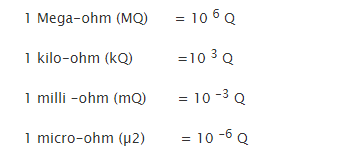Conductance (G)

It is termed as reciprocal of resistance.

A conductor’s resistance (example – uniform cross-sectional area of a wire) is mainly dependent on 4 factors.

Nature of material, denoted by p

Length, on whose variation a conductor’s resistance depends

Temperature of conductor

Cross section (A), whose variation is inversely dependent on A

Now,

R = P  l / A

Here P is resistivity or specific resistance.

Resistivity or specific resistance of a substance is expressed as the resistance which is present between opposite faces of hat substances’ meter cube.

Its unit is (Q-m) or ohm metre.

Conductivity (σ)

Specific resistance’s reciprocal regarding in any material is known as conductivity.

(σ = 1 /p) = Specific resistance

Conductivity = (σ = l x G / A)

Its unit is ohm/meter

Temperature’s effect on resistance

• Resistance regarding semiconductors likes electrolytes and carbon decrease with the increase in temperature. (In minus)
• Regarding metal conductors, its resistance increases with temperature rise. This increased rate is distinguishable for most metal which comes in the category of pure. This rate can be nearly about 1/1.50. This consideration is regarding total resistance in case of iron on centigrade

Temperature coefficient of resistance

It can be defined as change in resistance (/ohm) for a change in temperature (from 1°C to 0°C).

It can be stated as

V / I = constant or V /I = R

Here, a conductor’s resistance is denoted by R which is between the 2 considered points.

Also,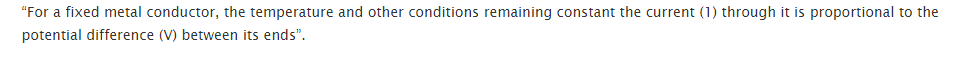Its linear relationship is not applicable to every non-metallic conductor like silicone carbide. Its relation stands to be at,

and

Voltage across individual resistance will remain to be similar, but its current will depend upon separate resistances’ value

Its equation is,

Rt = R[l + a(t – 20)]

This is possible when the expected temperature is beneath 20° C.

But in case of certain metals, it is essential for them to obtain zero magnetic induction and zero electrical resistance. This property is called superconductivity.

Few of the superconducting elements are:

• Mercury
• Zinc

Few of the superconducting alloys and compounds are:

• ZrC
• NbB
• NbN
• SnSb
• PbTh
• CuS
• PbAu

Temperature and Resistivity of Coefficient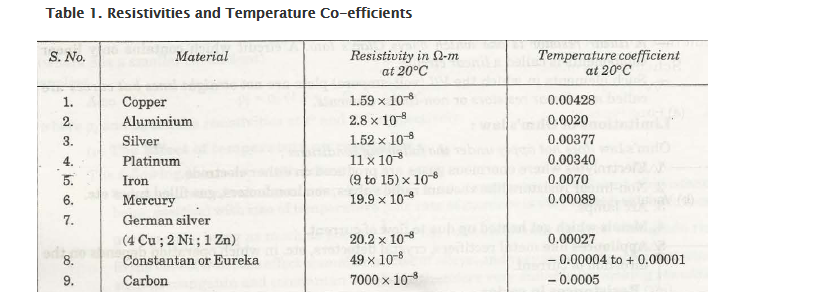1.5. Resistors

There are 2 main characteristics of resistors.

1. Wattage rating

The rating related to power may be high due to presence of several hundred watts. It can also be low to the range of 1/10 watt. Maximum wattage is indicated by Power rating which is regarding resistor. This can dissipate even without excessive heat.

1. Resistance

Availability regarding resistors from fraction of ohm to numerous mega ohms.

Links of Next Electrical Engineering Topics:-### Customer Reviews

My Homework Help
Rated 5.0 out of 5 based on 510 customer reviews at